# ISEE Upper Level Quantitative : Data Analysis

## Example Questions

### Example Question #51 : Data Analysis And Probability

Two fair dice are altered so that each has faces that read 1, 1, 1, 2, 2, 3. The two dice are rolled, and their sum is noted. Which is the greater quantity?

(a) The probability that the sum will be 3 or less

(b) The probability that the sum will be 4 or greater

It is impossible to tell from the information given.

(a) and (b) are equal.

(b) is greater.

(a) is greater.

(a) is greater.

Explanation:

A 3 or less can be rolled the following ways:

Both dice can be 1, for a roll of 2. This can happen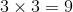ways, since each die has three 3's.

The first die can be 1 and the second die can be 2, for a roll of 3. This can happen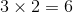ways, as each die has three 3's and two 2's.

Similarly, the first die can be 2 and the second die can be 3 - by symmetry, this can happen 6 ways.

This is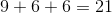outcomes out of 36 rolls that are favorable to the event, leaving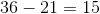unfavorable outcomes. This makes (a) greater.

### Example Question #51 : Data Analysis

The table below gives the population of Buchanan City in each census year from 1930  to 1980.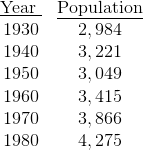No figures are given for 1965 but it is known that the population increased from 1960 to 1965 and from 1965 to 1970.

Which is the greater quantity?

(a) The population of Buchanan City in 1965

(b)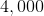It is impossible to tell from the information given.

(b) is greater.

(a) is greater.

(a) and (b) are equal.

(b) is greater.

Explanation:

Since the population increased over both the first and second half-decade, the population in 1965 had to be between the 1960 and 1970 levels - that is, it was between 3,415 and 3,866.

Therefore (b) is greater.

### Example Question #53 : Data Analysis And Probability

Two standard decks of fifty-two cards, which we will call Deck 1 and Deck 2, are presented to you. The aces have been removed from Deck 1 and mixed in with Deck 2. Which is the greater quantity?

(A) The probability that a card drawn randomly from Deck 1 is a spade

(B) The probability that a card drawn randomly from Deck 2 is a spade

(B) is greater

(A) and (B) are equal

(A) is greater

It is impossible to determine which is greater from the information given

(A) and (B) are equal

Explanation:

Four cards, including one spade (the ace of spades) changed decks.

Deck 1 now has forty-eight cards, including twelve spades. Therefore,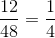of its cards are spades.

Deck 2 now has fifty-six cards, including fourteen spades. Therefore,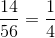of its cards are spades.

Therefore, the probability that a random card from either deck will be a spade isfor both decks.

### Example Question #51 : Data Analysis

A card is drawn at random from a standard 52-card deck. What are the odds against drawing either a king or a red three?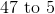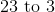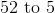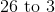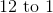Explanation:

There are four kings and two red threes, making six favorable outcomes and forty-six unfavorable ones. The odds against a favorable outcome are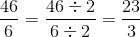or 23 to 3.

### Example Question #55 : Data Analysis And Probability

A card is drawn at random from a standard 52-card deck. The person drawing wins if the card he draws is a spade or an ace. What are the odds against drawing a winning card?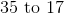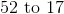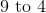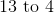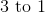Explanation:

Out of fifty-two cards, the winning cards are the thirteen spades and the three other aces - sixteen winning cards and thirty-six losing cards. The odds against drawing a winning card are therefore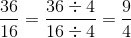or 9 to 4.

### Example Question #51 : Data Analysis

A standard deck of 52 cards is altered by the addition of two jokers. A card is drawn at random. What are the odds against drawing an ace, a king, or a joker?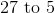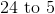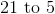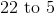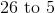Explanation:

The two jokers bring the deck to fifty-four cards; since there are four aces, four kings, and two jokers, there are ten favorable outcomes and forty-four unfavorable outcomes. This makes the odds against a favorable outcome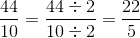or 22 to 5.

### Example Question #57 : Data Analysis And Probability

A card is drawn at random from a standard 52-card deck.

Which of the following outcomes has probability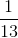?

Drawing a heart.

Drawing a black four or a black five.

Drawing a red face card (king, queen, jack).

Drawing an Old Maid card.

Drawing a black three.

Drawing a black four or a black five.

Explanation:

One thirteenth of 52 is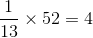so we are looking for an outcome that has four simple events - that is, four cards.

Drawing an Old Maid card: since a standard deck definitely does not have an Old maid card, this is incorrect.

Drawing a black three: there are two black threes, so this is not correct.

Drawing a red face card (king, queen, jack): This outcome includes two kings, two queens, and two jacks - six cards. This is not correct.

Drawing a heart: There are thirteen hearts, so this is not correct.

Drawing a black four or a black five: There are two black fours and two black fives - four cards. This is the correct response.

### Example Question #58 : Data Analysis And Probability

A standard 52-card deck is combined with the hearts and the spades from another deck, and the modified deck is shuffled. What is the probability that a card drawn at random from this modified deck will be a spade?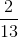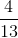Explanation:

The modified deck will have 78 cards (the original 52 plus the added 26), 26 of which will be spades (the original 13 plus the added 13). The probability of drawing a spade will therefore be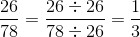### Example Question #59 : Data Analysis And Probability

A standard 52-card deck is combined with the diamonds and the clubs from another deck, and the modified deck is shuffled.

Which is the greater quantity?

(A) The probability that a card drawn at random from this modified deck will be an ace.

(B)(B) is greater

(A) is greater

(A) and (B) are equal

It is impossible to determine which is greater from the information given

(A) and (B) are equal

Explanation:

The modified deck will have 78 cards (the original 52 plus the added 26), 6 of which are aces (the original 4 plus the added ace of diamonds and ace of clubs); the probability that an ace will be drawn is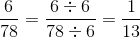The quantities are equal.

### Example Question #60 : Data Analysis And Probability

Two standard decks of fifty-two cards, which we will call Deck 1 and Deck 2, are presented to you. The kings have been removed from Deck 1 and mixed in with Deck 2. A card is then drawn at random from Deck 2.

What is the probability that the card will be a spade?None of the other responses gives the correct answer.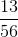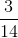None of the other responses gives the correct answer.

Explanation:

After the four kings - one of which is a spade - are transferred between decks, Deck 2 has fourteen spades out of fifty-six cards overall. This makes the probability of drawing a spade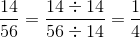.

This is not among the given choices, so the correct response is "none of these".

### All ISEE Upper Level Quantitative Resources